SAT Subject Math Level 1 Practice Test 6

Test Information20 questions24 minutes

Take more free SAT math 1&2 subject practice tests available from cracksat.net.

1.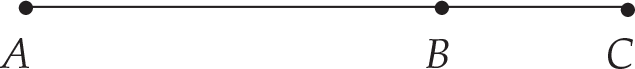Figure2

In Figure2, If the length of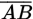is 2 more than 3 times the length of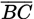, and AC = 14, what is the length of?

A. 2
B. 3
C. 4
D. 7
E. 12

2. Jean canpaint a house in 10 hours, and Dan can paint the same house in 12 hours.If Jean begins the job and does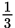of it and the n Dan takes over and finishes the job, what is the total time it takes the m to paint the house?

A. 10 hours, 40 minutes
B. 11 hours
C. 11 hours, 20 minutes
D. 11 hours, 40 minutes
E. 12 hours

3. If a= 4b, c = 8b2, and b ≠ 0, the n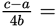A. -2b
B. 1 - 2b
C. 2b
D. 2b - 1
E. 2b + 3

4.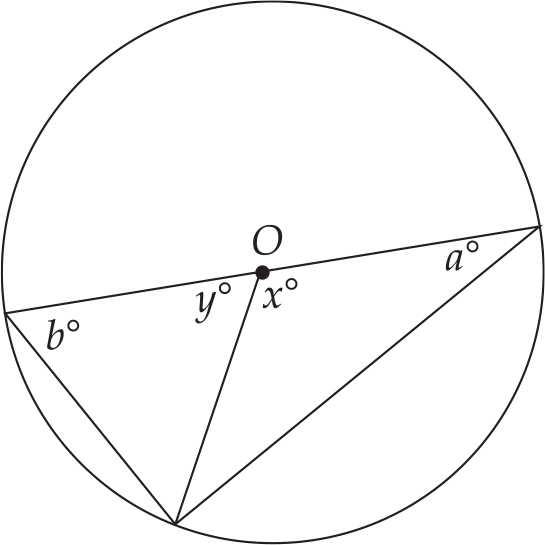Figure3

In Figure3, If O is the center of the circle, and the ratio of x toy is 2 to 1, what is the ratio of a to b?

A. 4:1
B. 3:1
C. 2:1
D. 1:2
E. 1:4

5. The cost of 2 sodas and 3 pretzels is \$4.60. If the cost of 2 pretzels is \$2.20,what is the cost of 2 sodas?

A. \$0.60
B. \$0.65
C. \$1.20
D. \$1.30
E. \$2.40

6. If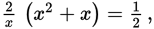the n x + 1 =

A.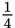B.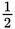C. 1
D.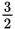E. 2

7.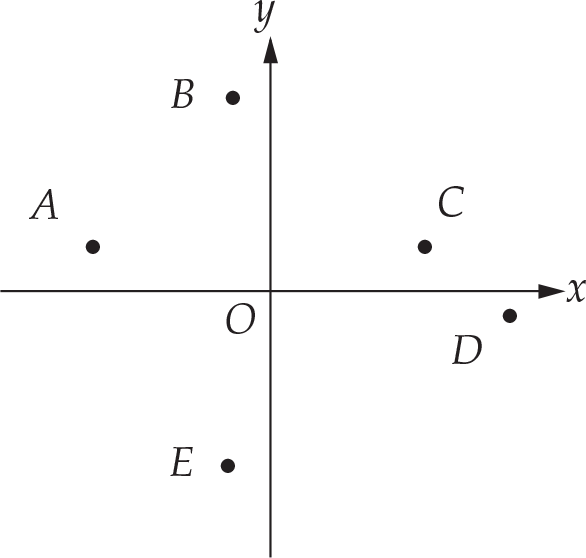Figure4

In Figure4, If 5 lines are drawn by connecting the labeled points with the origin, which of the lines would have the greatest slope?

A.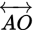B.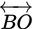C.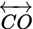D.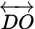E.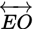8. If f(x) = 5x - x2, the nf(x) = 6 when x =

A. 1 only
B. 2 only
C. 1 or 5
D. 2 or 3
E. 2 or 6

9. If 25percent of a certain number is 36, what would 40 percent of the same number be?

A. 13.6
B. 22.5
C. 42.6
D. 52.8
E. 57.6

10.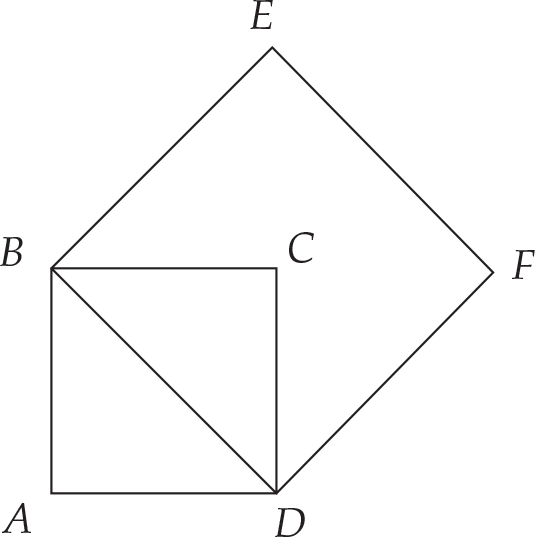Figure5

In Figure5, If the area of square ABCD is 5, what is the area of squareBEFD?

A. 7.07
B. 8.25
C. 10.00
D. 12.50
E. 25.00

11. If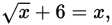the n x =

A. 4 only
B. 9 only
C. 4 or 9
D. -4 or 9
E. There is no solution.

12. A rubber ball is dropped from a height of 10 meters. If the ball always rebounds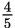the distance it has fallen, how far, in meters, will the ball have traveled at the moment it hits the ground for the fourth time?

A. 4.10
B. 5.12
C. 29.52
D. 43.92
E. 49.04

13. If avaries directly as b and If a = 4 when b = 5, whatis the value of a when b = 10?

A. 4
B. 5
C. 6
D. 8
E. 9

14. Which of the following figures has the greatest number of lines of symmetry?

A. Equilateral triangle
B. Rectangle
C. Square
D. Rhombus
E. Circle

15. If f(x) = x2 - 4x + 1,f(x) crosses the x-axis closest to which of the following points?

A. (-0.33, 0)
B. (0.27, 0)
C. (1.73, 0)
D. (3.27, 0)
E. (4.33, 0)

16.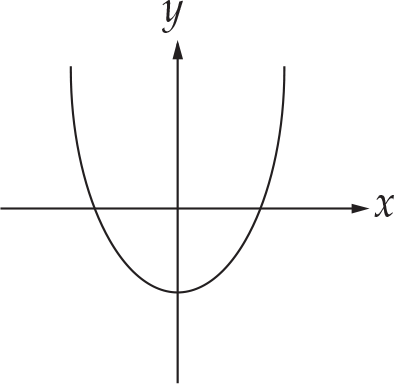Figure6

If the graph in Figure 6 represents f(x), which of the following graphs would represent | f(x) |?

A.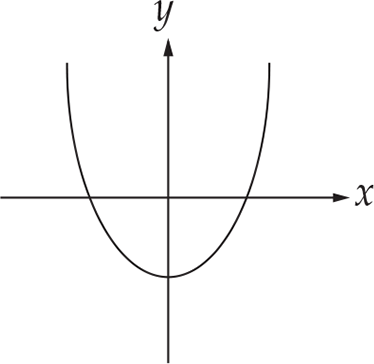B.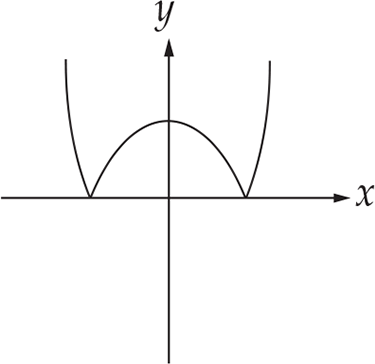C.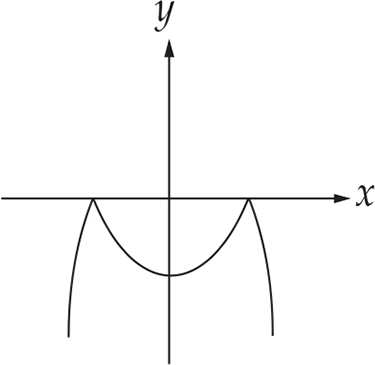D.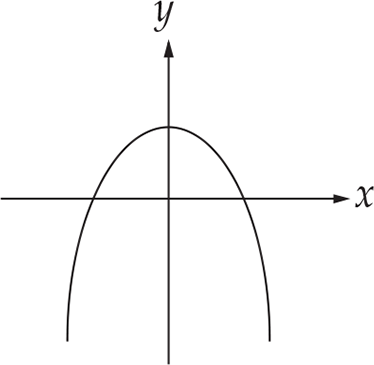E.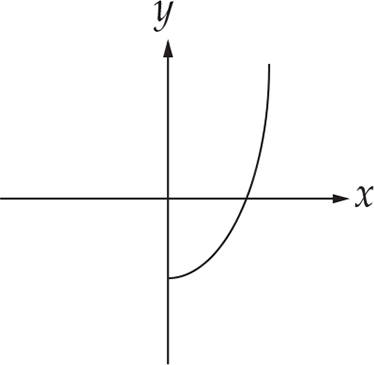17. If (x + y)2 = (x -y)2, which of the following must be true?

A. x = y
B. x = -y
C. x = 0
D. y = 0
E. x = 0 or y =0

18. Which of the following diagrams represents the solution set for y ≥2x + 3 and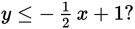A.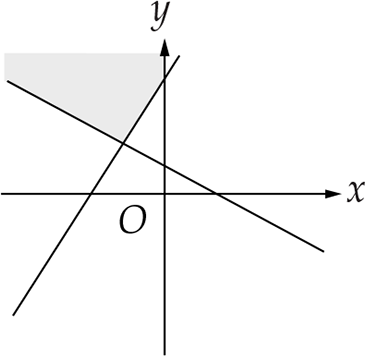B.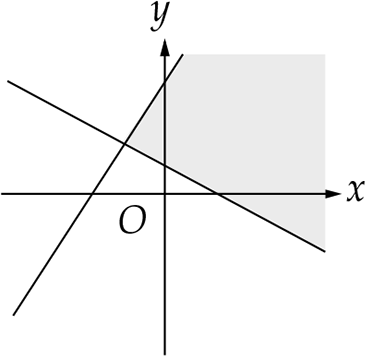C.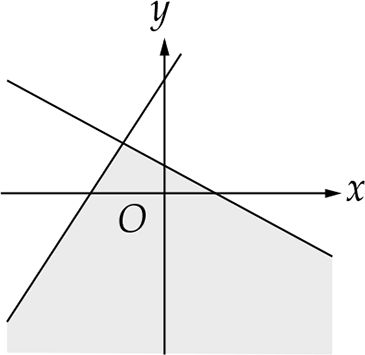D.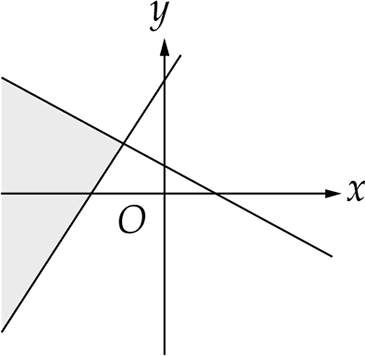E.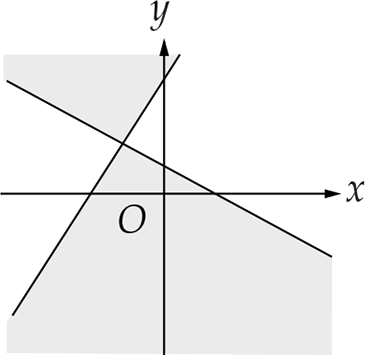19. If x#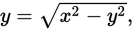the n 10 # (-6) =

A. 2
B. 8
C. 16
D. 32
E. 64

20.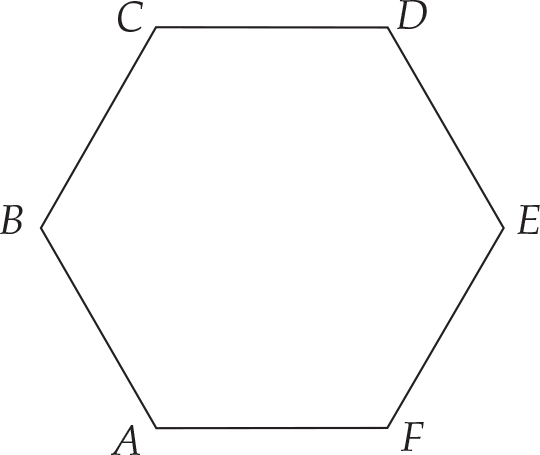Figure7

In Figure7, If ABCDEF is a regular hexagon, what is the approximate length of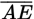If AB = 4?

A. 5.65
B. 5.73
C. 6.00
D. 6.93
E. 8.00

﻿